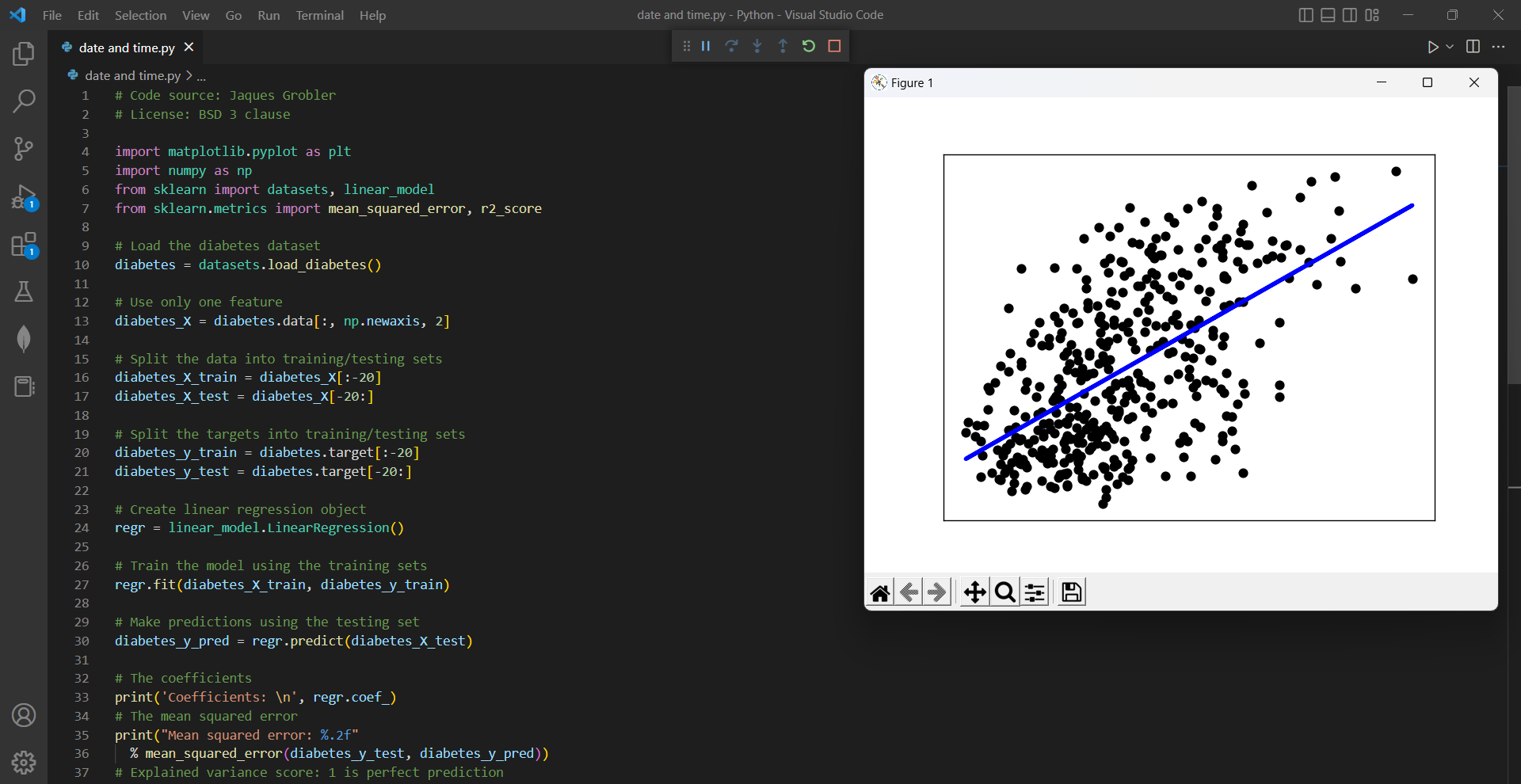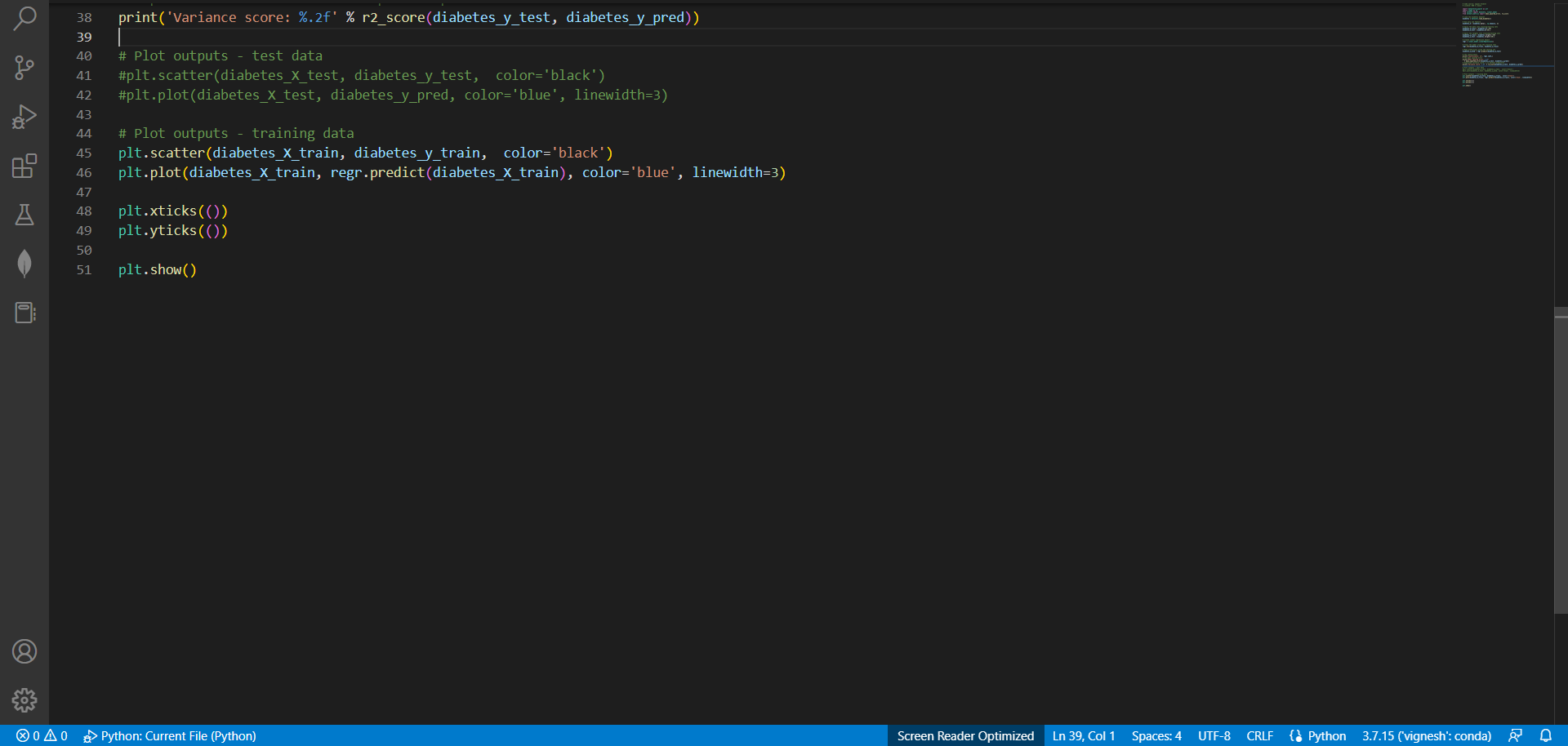# Get a perfect Regression Line in scikit learn,by vigneshchennai74Updated: Feb 15, 2023Solution Kit

The regression line is created using the linear regression algorithm from scikit-learn, which is a machine learning library for Python. The regression line shows the relationship between the input feature and the target variable and can be used to make predictions about the target variable given specific values of the input feature.

The regression line and linear regression are important tools that can be used to analyze and model the relationship between variables in a wide range of fields, helping us make better predictions, optimize processes and designs, and ultimately improve outcomes in various aspects of our lives.

Necessary libraries for performing linear regression analysis on a dataset using scikit-learn:

• matplotlib.pyplot is a plotting library used for creating visualizations of the data and regression line.
• numpy is a numerical computing library that provides support for array operations and linear algebra.
• datasets module from sklearn provides access to several popular datasets for practice purposes.
• linear_model module from sklearn contains the LinearRegression class that enables fitting a linear regression model to the data.
• mean_squared_error and r2_score are metrics from sklearn.metrics that are used to evaluate the performance of the linear regression model.

The regression line and the concept of linear regression are very helpful in many different areas of study and practice in this world. Like:

• Marketing and advertising: Regression analysis can be used to model the relationship between advertising spending and sales, as well as to identify which factors have the greatest impact on consumer behavior. This information is used to optimize marketing campaigns and increase sales.
• Medicine and healthcare: Regression analysis is often used to model the relationship between different health outcomes and various risk factors such as age, weight, and lifestyle choices. This information can be used to develop more effective prevention and treatment strategies.

Here is the example of how to plot the perfect regression line using Sk-learn:Preview of the output that you will get on running this code from your IDE

### Code

In this solution we have used Scikit learn Library

1. Copy the code using the "Copy" button above, and paste it in a Python file in your IDE.
2. Run the file to get a perfect regression line

I hope you found this useful. I have added the link to dependent libraries, version information in the following sections.

I found this code snippet by searching for "How to i get a perfect Regression Line in Scikit learn" in kandi. You can try any such use case!

### Dependent Library

scikit-learnby scikit-learn

Python53431Version:1.2.2scikit-learn: machine learning in Python

Support
Quality
Security
Reuse

scikit-learnby scikit-learn

Python53431Version:1.2.2License: Permissive (BSD-3-Clause)

scikit-learn: machine learning in Python
Support
Quality
Security
Reuse

If you do not have Scikit-learn that is required to run this code, you can install it by clicking on the above link and copying the pip Install command from the scikit learn page in kandi.

You can search for any dependent library on kandi like Scikit-learn

### Environment Test

I tested this solution in the following versions. Be mindful of changes when working with other versions.

1. The solution is created in Python 3.7.15 version
2. The solution is tested on scikit-learn 1.0.2 version

Using this solution, we are able to get a perfect regression line using Scikit learn library in Python with simple steps. This process also facilities an easy to use, hassle free method to create a hands-on working version of code which would help us get a perfect line in Python.

### Support

1. For any support on kandi solution kits, please use the chat
2. For further learning resources, visit the Open Weaver Community learning page.

See similar Kits and Libraries